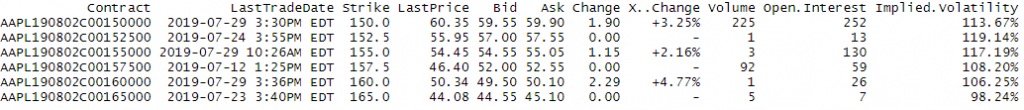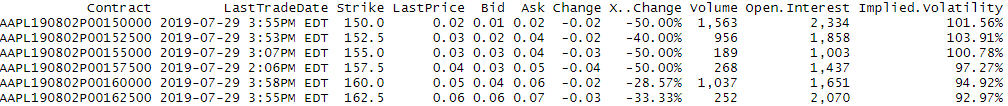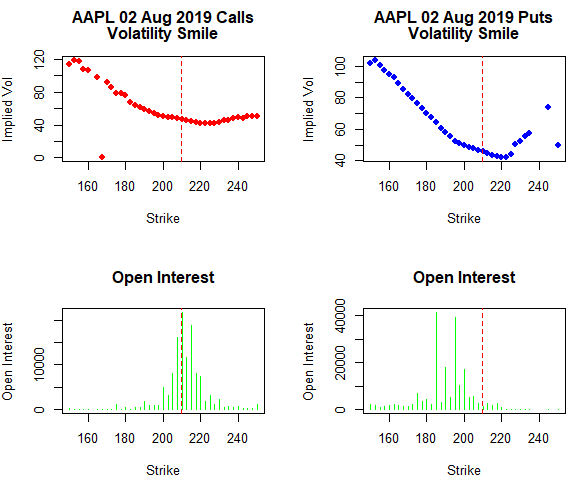# Plotting Volatility Smile in R

The main flaw of the Black Scholes model is that it assumes that the volatility of options contracts is constant for different strike prices. This assumption is not reflected in the real world where different strikes prices have different Implied Volatility values showing that investors and traders assign higher premiums for options that allow them to protect their portfolios.

Usually, the implied volatility values form a convex curve along the strike prices. This curve is called the Volatility Smile. This smile shape is formed because the market establishes higher values of implied volatility for in-the-money (ITM) and out-of-money (OTM) options compared to the implied volatility of the at-the -money (ATM) options contracts, that have lower values of implied volatility.

Some of the causes that explain this are the supply and demand of options from investors that demand high put contracts to protect their portfolios or traders and speculators that set strikes prices near resistance levels.

We would use two csv files with options data for call and put contracts for AAPL symbol and plot the volatility smile using R.

AAPL puts

AAPL calls

# Get the two files that have the data for calls and puts at expiration date 02 August 2019. The source of these files is Yahoo Finance. https://finance.yahoo.com/quote/SPY/options

calls <- read.csv(C:/Users/Nicolas/Documents/Derivatives with R/Volatility Smile/AAPL_calls.csv, stringsAsFactors = FALSE)

puts <- read.csv("C:/Users/Nicolas/Documents/Derivatives with R/Volatility Smile/AAPL_puts.csv ",stringsAsFactors = FALSE)

head(calls)head(puts)# The next step is to plot the Implied Volatility and Strike Prices and then Open Interest by Strike Prices. First we have to clean the data as the Implied Volatility column is a character column. We will remove the '%' and convert to number

calls$Implied.Volatility <- as.numeric(gsub('%','',calls$Implied.Volatility))

puts$Implied.Volatility <- as.numeric(gsub('%','',puts$Implied.Volatility))

# Change the ',' with empty character as R does not interpret ',' as thousands values

calls$Open.Interest <- as.numeric(gsub(',','',calls$Open.Interest))

puts$Open.Interest <- as.numeric(gsub(',','',puts$Open.Interest))

# Total of 4 graphs showing Implied Volatility and Open Interest patterns among Strikes Prices.

S = 209.68

par(mfrow=c(2,2))

#Plot Implied Volatility vs Strike Prices

plot(calls$Strike, calls$Implied.Volatility, type="p", col="red", pch = 19,
main=c("AAPL 02 Aug 2019 Calls", "Volatility Smile"), xlab="Strike", ylab="Implied Vol")

abline(v=S, col="red", lty=2)

plot(puts$Strike, puts$Implied.Volatility, type="p", col="blue",pch = 19,     main=c("AAPL 02 Aug 2019 Puts", "Volatility Smile"), xlab="Strike", ylab="Implied Vol")

abline(v=S, col="red", lty=2)

#Plot Open Interest along Strike Prices

plot(calls$Strike, calls$Open.Interest, type="h",pch = 19, col='green',     main="Open Interest", xlab="Strike", ylab="Open Interest")

abline(v=S, col="red", lty=2)

plot(puts$Strike, puts$Open.Interest, type="h",pch = 19, col='green',     main="Open Interest", xlab="Strike", ylab="Open Interest")

abline(v=S, col="red", lty=2)


Volatility Smile-Open Interest AAPL optionsThe red vertical lines mark the underlying stock prices for AAPL on 29th July 2019 in each graph. It is possible to observe the smile shape in the Implied Volatility vs Strikes Prices. This pattern is more pronounced for puts options. Also there is a high demand for puts options contracts that are out-the-money (below the Underlying price) as is observed in the Open Interest right chart. This insight is reflected by the demand for put options to protect portfolios.